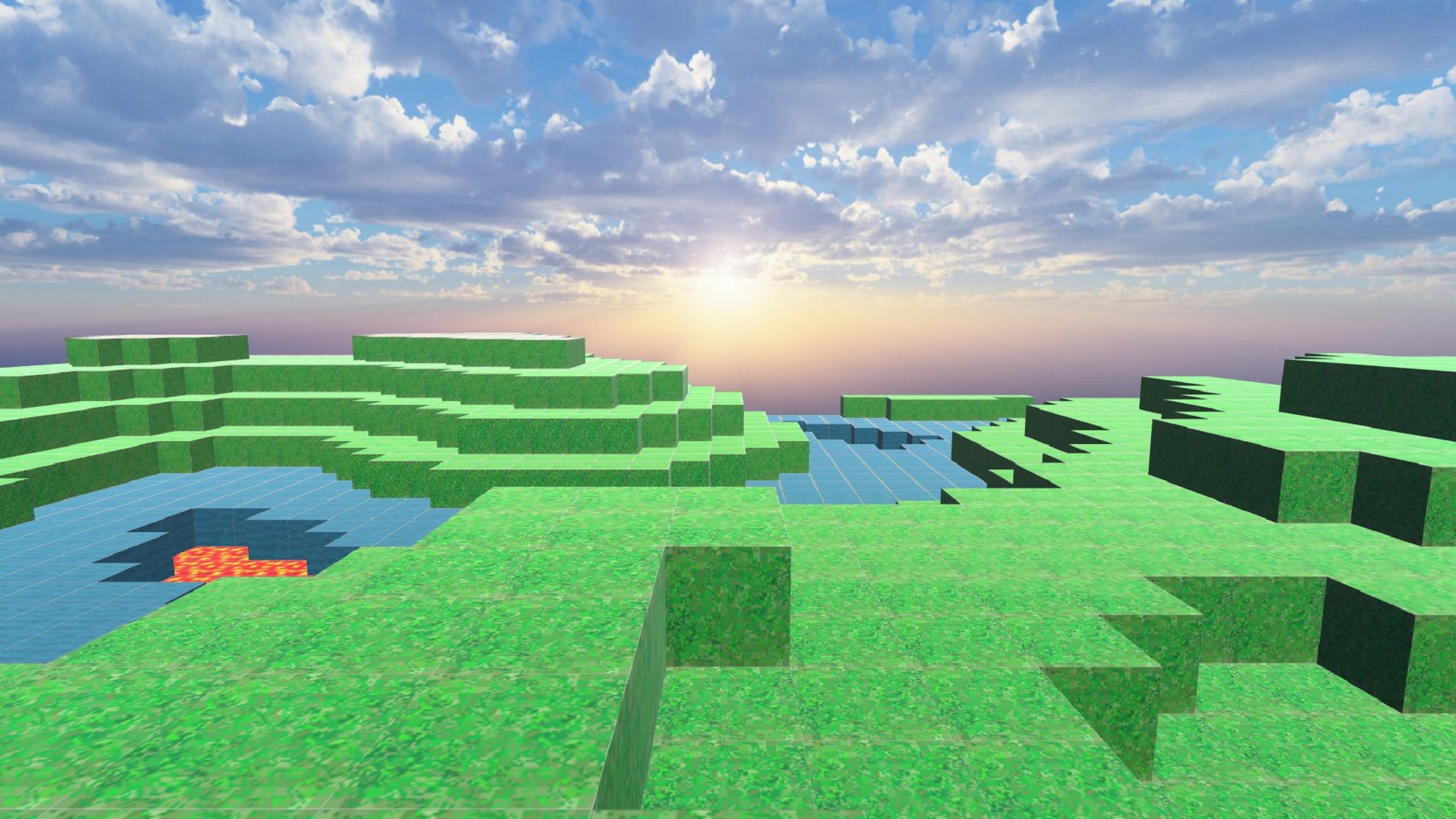# Creating a Minecraft-like landscape and uploading to STYLY -part 1 of 2-

Creating a Minecraft-like landscape and uploading to STYLY -part 1 of 2-

In this article, I will introduce how to create a Minecraft-like landscape and upload it to STYLY.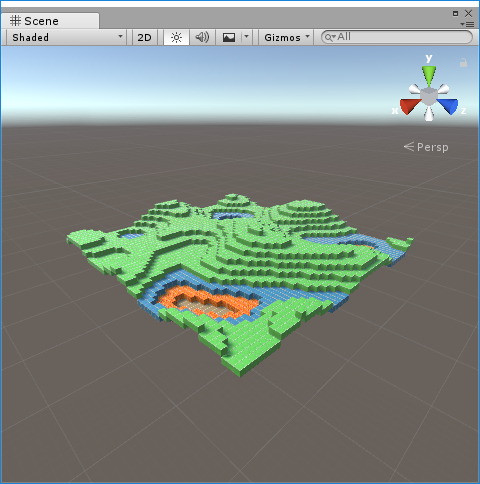Minecraft-like landscape

## Confirm sample assets with WebEditor

Confirm sample assets with WebEditor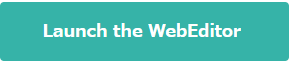* If you  register STYLY, you can check the sample assets created with this TIPS with WebEditor.
If you do not register an account, please register again and click this button again.

Register for account from here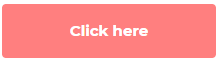## Sample

Open a sample scene from the STYLY GALLERY.
Sample scene

## How to create a Minecraft-like landscape

We will create a Minecraft-like landscape using the following 5 steps.

• Auto-creating a landscape in Unity
• Pasting textures
• Combining meshes and improving performance
• Save auto-created meshes

I will explain the 5 steps briefly.

### Auto-creating a landscape in Unity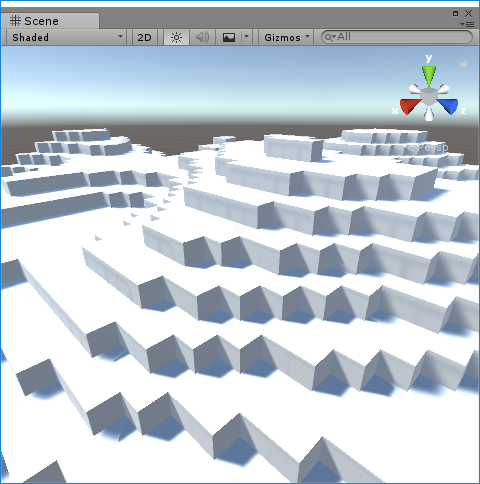Use the random number generator and place cubes so they form a landscape.

### Pasting textures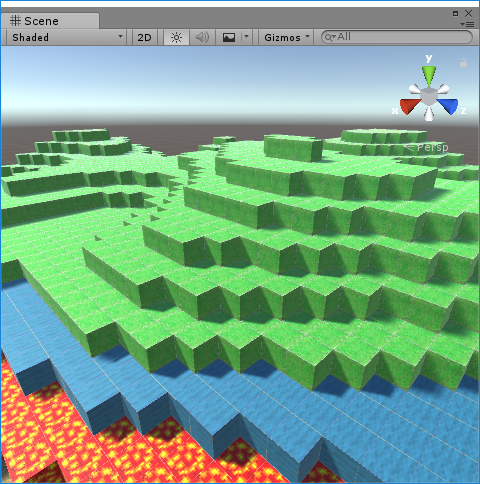Paste textures to the cubes and change the texture according to the height of the cube.

### Combining meshes and improving performance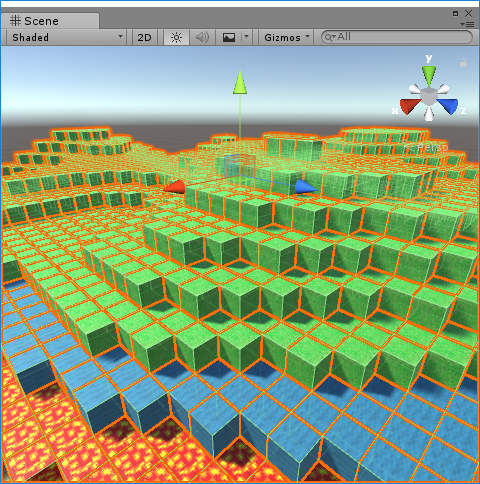If we upload 2500 cubes to STYLY, the viewer will freeze.By combining the meshes of 2500 cubes, the project will not freeze even when uploaded to STYLY.

### Save auto-created meshes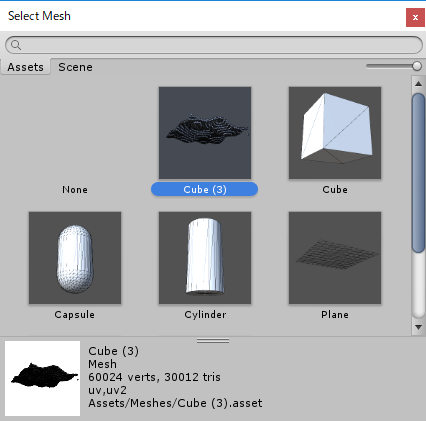Save the created meshPaste the created mesh to a game object, and create a prefab from it.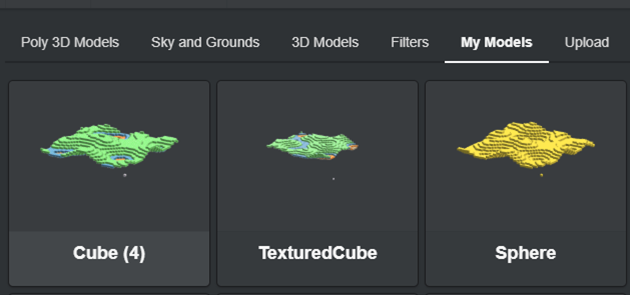Ok, let’s go through the steps in detail.

## Auto-creating a landscape in Unity

### Preparation

• Creating a base object

Create a cube to the scene, and name it “Ground”.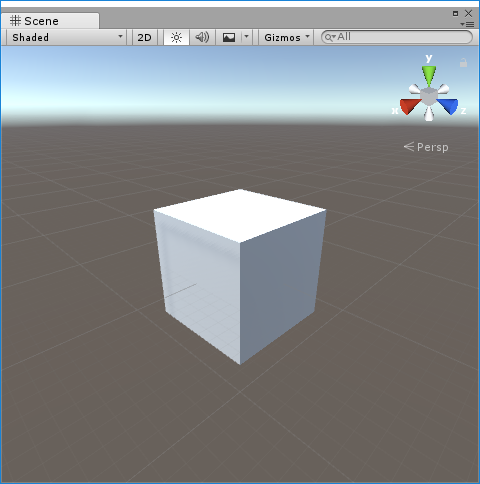Creating the Ground object

• Create a ground forming object

Add the following script and name it “VoxelGround.cs”.

``````using UnityEngine;

public class VoxelGround : MonoBehaviour
{
private float sizeX = 50f;
private float sizeY = 10f;
private float sizeZ = 50f;
private float sizeW = 17f;
private void Awake()
{
var material = this.GetComponent<MeshRenderer>().material;
for (float x = 0; x < sizeX; x++)
{
for (float z = 0; z < sizeZ; z++)
{
GameObject cube = GameObject.CreatePrimitive(PrimitiveType.Cube);
cube.transform.SetParent(transform);
float noise = Mathf.PerlinNoise(x/ sizeW, z/ sizeW);
float y = Mathf.Round(sizeY * noise);
cube.transform.localPosition = new Vector3(x, y, z);
}
}
transform.localPosition = new Vector3(-sizeX / 2, 0, -sizeZ / 2);
}
}``````

### Confirm the results

Add “VoxelGround” as a component to Ground object.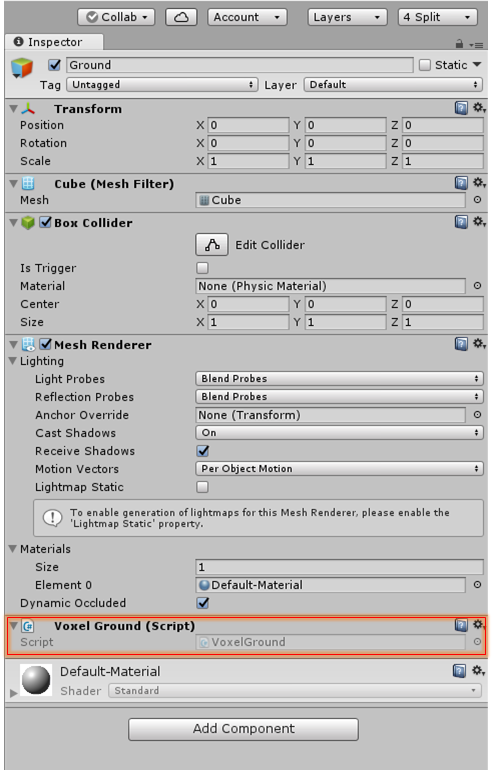Press the Ctrl + P keys and if a landscape like the one in the image below gets created, it is a success.Success

### Explanation

``````    private float sizeX = 50f;　//The number of blocks to the X-axis
private float sizeY = 10f;　//The max height of the landscape
private float sizeZ = 50f;　//The number of blocks to the Z-axis
private float sizeW = 17f;　//The size of ``````

We specify the size of the landscape here.

The sizeW might be hard to understand, but it is the distance from the top of the mountain to the ground.

So, in the sample, a mountain is created from 17 blocks, which means there will be about 3 mountains created from 50 blocks.

If we make the number smaller than 17, there will be more mountains created in the scene.

``````    private void Awake()
{
var material = this.GetComponent<MeshRenderer>().material;
for (float x = 0; x < sizeX; x++)
{
for (float z = 0; z < sizeZ; z++)
{
//Creating blocks
GameObject cube = GameObject.CreatePrimitive(PrimitiveType.Cube);
cube.transform.SetParent(transform);

//Create a variable that smoothly changes according to the X-axis and Y-axis
float noise = Mathf.PerlinNoise(x/ sizeW, z/ sizeW);

//Set the number as the Y-axis position (height)
float y = Mathf.Round(sizeY * noise);
cube.transform.localPosition = new Vector3(x, y, z);
}
}
transform.localPosition = new Vector3(-sizeX / 2, 0, -sizeZ / 2);
}``````

In the sample, we will create 50 blocks on the X-axis, and 50 blocks on the Z-axis, resulting in 2500 blocks.

By using Mathf.PerlinNoise as the height of the blocks, we can create a smooth landscape.

## Pasting textures

### Preparation

• Importing textures

detailed minecraft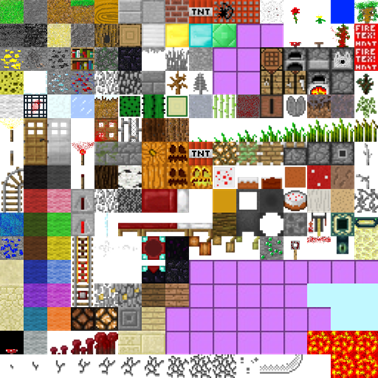Texture pack

Import the texture to the Unity editor.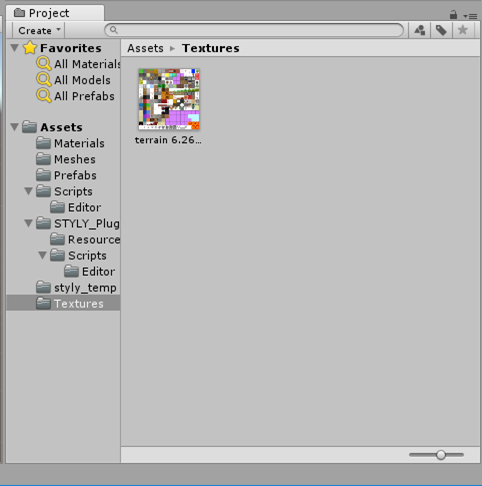Importing the texture

• Creating a material

Drag and drop the imported texture to the “Ground” game object.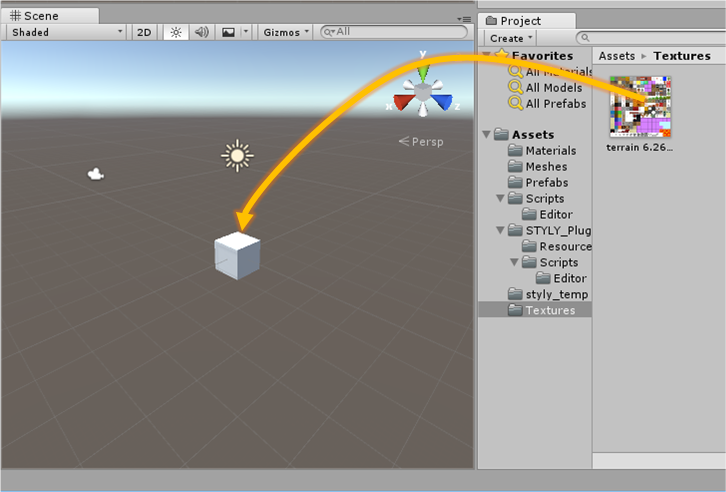Drag and drop the texture to the game object

The texture will be applied to the “Ground” object, and check that a material with the same name as the texture is created.

( In the sample, the material name is “terrain 6.26.56 PM” )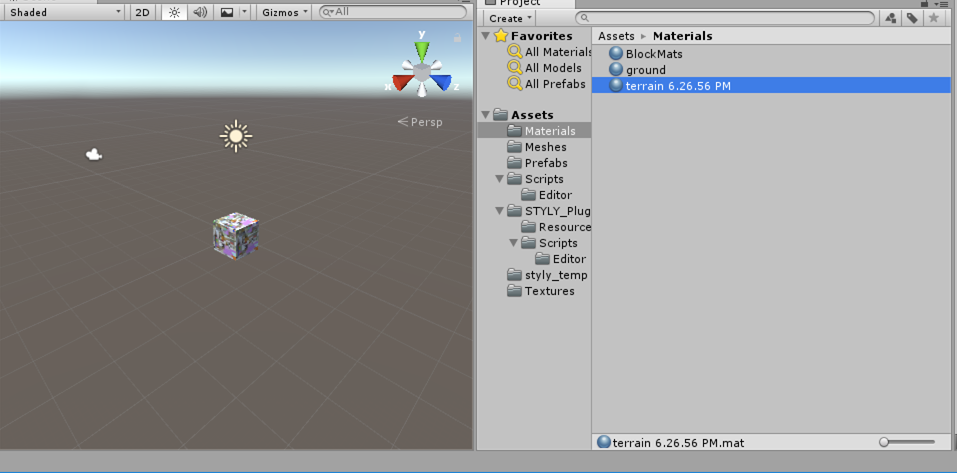A material is created

• Adjusting a ground forming object

```using UnityEngine;

public class VoxelGround : MonoBehaviour
{

private float sizeX = 50f;
private float sizeY = 10f;
private float sizeZ = 50f;
private float sizeW = 17f;

private void Awake()
{
var material = this.GetComponent<MeshRenderer>().material;
for (float x = 0; x < sizeX; x++)
{
for (float z = 0; z < sizeZ; z++)
{
GameObject cube = GameObject.CreatePrimitive(PrimitiveType.Cube);
cube.transform.SetParent(transform);
cube.GetComponent<MeshRenderer>().material = material;

float noise = Mathf.PerlinNoise(x/ sizeW, z/ sizeW);
float y = Mathf.Round(sizeY * noise);
cube.transform.localPosition = new Vector3(x, y, z);

SetUV(cube);
}
}
transform.localPosition = new Vector3(-sizeX / 2, 0, -sizeZ / 2);
}

private void SetUV(GameObject cube)
{
cube.GetComponent<MeshFilter>().mesh.uv = GetBlockUVs(2, 15);
if (cube.transform.position.y > sizeY * 0.3f)
{
cube.GetComponent<MeshFilter>().mesh.uv = GetBlockUVs(0, 15);
}
else if (cube.transform.position.y > sizeY * 0.2f)
{
cube.GetComponent<MeshFilter>().mesh.uv = GetBlockUVs(1, 2);
}
else if (cube.transform.position.y > sizeY * 0.1f)
{
cube.GetComponent<MeshFilter>().mesh.uv = GetBlockUVs(15, 0);
}
}

private Vector2[] GetBlockUVs(float tileX, float tileY)
{
float pixelSize = 16;
float tilePerc = 1 / pixelSize;

float umin = tilePerc * tileX;
float umax = tilePerc * (tileX + 1);
float vmin = tilePerc * tileY;
float vmax = tilePerc * (tileY + 1);

Vector2[] blockUVs = new Vector2;

//-X
blockUVs = new Vector2(umax, vmax);
blockUVs = new Vector2(umin, vmax);
blockUVs = new Vector2(umax, vmin);
blockUVs = new Vector2(umin, vmin);

//+Y
blockUVs = new Vector2(umin, vmin);
blockUVs = new Vector2(umax, vmin);
blockUVs = new Vector2(umin, vmax);
blockUVs = new Vector2(umax, vmax);

//-Z
blockUVs = new Vector2(umax, vmin);
blockUVs = new Vector2(umin, vmax);
blockUVs = new Vector2(umin, vmin);
blockUVs = new Vector2(umax, vmax);

//+Z
blockUVs = new Vector2(umax, vmin);
blockUVs = new Vector2(umin, vmax);
blockUVs = new Vector2(umin, vmin);
blockUVs = new Vector2(umax, vmax);

//-Y
blockUVs = new Vector2(umax, vmin);
blockUVs = new Vector2(umin, vmax);
blockUVs = new Vector2(umin, vmin);
blockUVs = new Vector2(umax, vmax);

//+X
blockUVs = new Vector2(umin, vmin);
blockUVs = new Vector2(umax, vmin);
blockUVs = new Vector2(umin, vmax);
blockUVs = new Vector2(umax, vmax);

return blockUVs;
}
}```

### Confirm the result

Press the Ctrl + P keys, and see if a landscape like the one in the next image is created.Apply textures to the landscape

### ExplanationTexture pack

The image above is called a texture pack, and the textures needed to create a minecraft-like landscape is included.

By using a texture pack, we only need one material for the scene, so we are able to combine the meshes.

```    private void SetUV(GameObject cube)
{
cube.GetComponent<MeshFilter>().mesh.uv = GetBlockUVs(2, 15);
if (cube.transform.position.y > sizeY * 0.3f)
{
cube.GetComponent<MeshFilter>().mesh.uv = GetBlockUVs(0, 15);　//山
}
else if (cube.transform.position.y > sizeY * 0.2f)
{
cube.GetComponent<MeshFilter>().mesh.uv = GetBlockUVs(1, 2);　//water surface
}
else if (cube.transform.position.y > sizeY * 0.1f)
{
cube.GetComponent<MeshFilter>().mesh.uv = GetBlockUVs(15, 0);　//lava
}
}```

In the sample, the texture is changed according to the height of the landscape.

We set the following:

For GetBlockUVs(0, 15) we set the left top texture of green grass

For GetBlockUVs(15, 0) we set the right bottom texture of red lava

```    private Vector2[] GetBlockUVs(float tileX, float tileY)
{

float pixelSize = 16;
float tilePerc = 1 / pixelSize;

float umin = tilePerc * tileX;
float umax = tilePerc * (tileX + 1);
float vmin = tilePerc * tileY;
float vmax = tilePerc * (tileY + 1);

Vector2[] blockUVs = new Vector2;

//-X
blockUVs = new Vector2(umax, vmax);
blockUVs = new Vector2(umin, vmax);
blockUVs = new Vector2(umax, vmin);
blockUVs = new Vector2(umin, vmin);

//+Y
blockUVs = new Vector2(umin, vmin);
blockUVs = new Vector2(umax, vmin);
blockUVs = new Vector2(umin, vmax);
blockUVs = new Vector2(umax, vmax);

//-Z
blockUVs = new Vector2(umax, vmin);
blockUVs = new Vector2(umin, vmax);
blockUVs = new Vector2(umin, vmin);
blockUVs = new Vector2(umax, vmax);

//+Z
blockUVs = new Vector2(umax, vmin);
blockUVs = new Vector2(umin, vmax);
blockUVs = new Vector2(umin, vmin);
blockUVs = new Vector2(umax, vmax);

//-Y
blockUVs = new Vector2(umax, vmin);
blockUVs = new Vector2(umin, vmax);
blockUVs = new Vector2(umin, vmin);
blockUVs = new Vector2(umax, vmax);

//+X
blockUVs = new Vector2(umin, vmin);
blockUVs = new Vector2(umax, vmin);
blockUVs = new Vector2(umin, vmax);
blockUVs = new Vector2(umax, vmax);

return blockUVs;
}```

In the sample, we set the texture to the cube-shaped meshes.

For pixelSize, we set the row number of the texture pack. In the sample, we are using a 16 x 16 texture pack, so I set the variable as 16.

I referred to the below blog for UV.

”Unity の Cube を使う時、側面の天地が揃う UV 作った”

## Conclusion

That will be all for part1.

We have completed the first 2 steps of the whole 5 steps, and we have finished creating the appearance of the landscape.

• Auto-creating a landscape in Unity
• Pasting textures
• Combining meshes and improving performance
• Save auto-created meshes